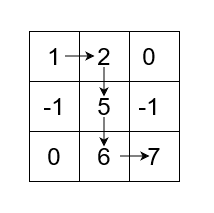# Clearing the Forest

Posted: 11 Mar, 2021
Difficulty: Moderate

## PROBLEM STATEMENT

#### Ninja lives in a beautiful city known as Byteland. A grand festive event is going to be organised in the city. To make place for the event, King has ordered Ninja to clear the nearby forest. The forest can be represented in the form of ‘N’*‘M’ grid ‘FOREST’, where each cell of ‘FOREST’ can have one of the possible values:

``````0 -> The cell is empty and Ninja can pass through it.
‘X’  -> The cell contains a tree with a height equal to ‘X’ where  ‘X’ > 0 and Ninja can pass through it.
-1 -> The cell can not be visited by Ninja.
``````

#### For example :

``````If ‘FOREST’ is :
[1,2,0]
[-1,5,-1]
[0,6,7]

We can see we need 4 steps to cut down all trees as shown below:
````````````So, the output will be 4.
``````

#### Input Format:

``````The first line of input contains an integer ‘T’ denoting the number of test cases. Then each test case follows.

The first line of each test case contains two single space-separated integers ‘N’ and ‘M’ representing the number of rows and columns in ‘FOREST’.

The next ‘N’ lines of each test case contain ‘M’ single space-separated integers denoting the values of ‘FOREST’.
``````

#### Output format:

``````For each test case, print a single line containing a singe integer representing the minimum number of steps to cut down all the trees.

The output of every test case will be printed in a separate line.
``````

#### Note :

``````You don’t have to print anything, it has already been taken care of. Just implement the given function.
``````

#### Constraints

``````1 <= T <=10
1 <= N, M <= 50
-1 <= FOREST[i] <= 10 ^ 5

Where ‘T’ denotes the number of test cases, ‘N’ denotes the number of rows and ‘M’ denotes the number of columns of ‘FOREST’.

Time limit: 1 second
``````Approach 1

The idea is to first find all the trees and their coordinates and then sort the trees according to their height. Then we just need to find the steps needed between two consecutive trees. We will use Breadth-First-Search algorithm to find the shortest path between two trees.

Complete Algorithm:

1. Iterate over ‘FOREST’ and find out all trees (i.e cells having value greater than 0).
2. Save height and the coordinates of all trees in an array/list of arrays/lists say ‘TREES’ and sort the array according to heights (We can also use HashMap for this step).
3. Make a helper function say FIND_DIST() which receives four variables that are the coordinates of starting point and ending point for which we want to find the distance between them.
4. FIND_DIST() will use BFS to find distance between points as explained below:
1. Let’s say (‘R1’, ’C1’) are coordinates of starting point and (‘R2, ‘C2’) are coordinates for ending point.
2. We will use a queue to implement BFS.
3. Intialise an integer variable ‘STEP’ = 0.
4. Enque the starting point coordinates or we can say (‘R1’, ’C1’) .
5. Repeat steps 6 and 7 till the queue is not empty.
6. Increase the value of ‘STEP’ by 1.
7. Let’s say ‘SIZE’ is the size of queue, so run a for loop ‘SIZE’ times and inside the loop do following steps:
1. Check all the four neighbours(if possible) of current coordinates.
2. If any of them match with (‘R2, ‘C2’) then return ‘STEP’.
3. Enque the neighbours which are possible to pass through and are not visited till.
5. Iterate over “TREES’ and find dist between two consecutive trees with help of FIND_DIST() function.
6. If at any step FIND_DIST() returns -1 in the earlier step then we will also return -1. Otherwise we will return the sum of all values returned by FIND_DIST().